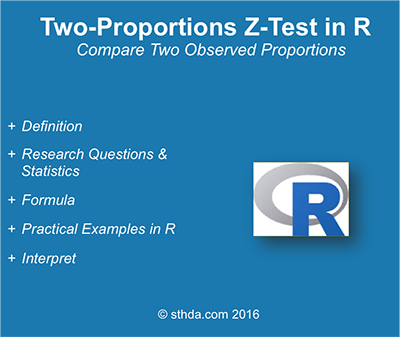# What is two-proportions z-test?

The two-proportions z-test is used to compare two observed proportions. This article describes the basics of two-proportions *z-test and provides pratical examples using R sfoftware**.

For example, we have two groups of individuals:

• Group A with lung cancer: n = 500
• Group B, healthy individuals: n = 500

The number of smokers in each group is as follow:

• Group A with lung cancer: n = 500, 490 smokers, $$p_A = 490/500 = 98%$$
• Group B, healthy individuals: n = 500, 400 smokers, $$p_B = 400/500 = 80%$$

In this setting:

• The overall proportion of smokers is $$p = frac{(490 + 400)}{500 + 500} = 89%$$
• The overall proportion of non-smokers is $$q = 1-p = 11%$$

We want to know, whether the proportions of smokers are the same in the two groups of individuals?# Research questions and statistical hypotheses

Typical research questions are:

1. whether the observed proportion of smokers in group A ($$p_A$$) is equal to the observed proportion of smokers in group ($$p_B$$)?
2. whether the observed proportion of smokers in group A ($$p_A$$) is less than the observed proportion of smokers in group ($$p_B$$)?
3. whether the observed proportion of smokers in group A ($$p_A$$) is greater than the observed proportion of smokers in group ($$p_B$$)?

In statistics, we can define the corresponding null hypothesis ($$H_0$$) as follow:

1. $$H_0: p_A = p_B$$
2. $$H_0: p_A \leq p_B$$
3. $$H_0: p_A \geq p_B$$

The corresponding alternative hypotheses ($$H_a$$) are as follow:

1. $$H_a: p_A \ne p_B$$ (different)
2. $$H_a: p_A > p_B$$ (greater)
3. $$H_a: p_A < p_B$$ (less)

Note that:

• Hypotheses 1) are called two-tailed tests
• Hypotheses 2) and 3) are called one-tailed tests

# Formula of the test statistic

## Case of large sample sizes

The test statistic (also known as z-test) can be calculated as follow:

$z = \frac{p_A-p_B}{\sqrt{pq/n_A+pq/n_B}}$

where,

• $$p_A$$ is the proportion observed in group A with size $$n_A$$
• $$p_B$$ is the proportion observed in group B with size $$n_B$$
• $$p$$ and $$q$$ are the overall proportions
• if $$|z| < 1.96$$, then the difference is not significant at 5%
• if $$|z| \geq 1.96$$, then the difference is significant at 5%
• The significance level (p-value) corresponding to the z-statistic can be read in the z-table. We’ll see how to compute it in R.

Note that, the formula of z-statistic is valid only when sample size ($$n$$) is large enough. $$n_Ap$$, $$n_Aq$$, $$n_Bp$$ and $$n_Bq$$ should be $$\geq$$ 5.

## Case of small sample sizes

The Fisher Exact probability test is an excellent non-parametric technique for comparing proportions, when the two independent samples are small in size.

# Compute two-proportions z-test in R

## R functions: prop.test()

The R functions prop.test() can be used as follow:

prop.test(x, n, p = NULL, alternative = "two.sided",
correct = TRUE)

• x: a vector of counts of successes
• n: a vector of count trials
• alternative: a character string specifying the alternative hypothesis
• correct: a logical indicating whether Yates’ continuity correction should be applied where possible

Note that, by default, the function prop.test() used the Yates continuity correction, which is really important if either the expected successes or failures is < 5. If you don’t want the correction, use the additional argument correct = FALSE in prop.test() function. The default value is TRUE. (This option must be set to FALSE to make the test mathematically equivalent to the uncorrected z-test of a proportion.)

## Compute two-proportions z-test

We want to know, whether the proportions of smokers are the same in the two groups of individuals?

res <- prop.test(x = c(490, 400), n = c(500, 500))
# Printing the results
res 

2-sample test for equality of proportions with continuity correction
data:  c(490, 400) out of c(500, 500)
X-squared = 80.909, df = 1, p-value < 2.2e-16
alternative hypothesis: two.sided
95 percent confidence interval:
0.1408536 0.2191464
sample estimates:
prop 1 prop 2
0.98   0.80 

The function returns:

• the value of Pearson’s chi-squared test statistic.
• a p-value
• a 95% confidence intervals
• an estimated probability of success (the proportion of smokers in the two groups)

Note that:

• if you want to test whether the observed proportion of smokers in group A ($$p_A$$) is less than the observed proportion of smokers in group ($$p_B$$), type this:
prop.test(x = c(490, 400), n = c(500, 500),
alternative = "less")
• Or, if you want to test whether the observed proportion of smokers in group A ($$p_A$$) is greater than the observed proportion of smokers in group ($$p_B$$), type this:
prop.test(x = c(490, 400), n = c(500, 500),
alternative = "greater")

## Interpretation of the result

The p-value of the test is 2.36310^{-19}, which is less than the significance level alpha = 0.05. We can conclude that the proportion of smokers is significantly different in the two groups with a p-value = 2.36310^{-19}.

Note that, for 2 x 2 table, the standard chi-square test in chisq.test() is exactly equivalent to prop.test() but it works with data in matrix form.

The result of prop.test() function is a list containing the following components:

• statistic: the number of successes
• parameter: the number of trials
• p.value: the p-value of the test
• conf.int: a confidence interval for the probability of success.
• estimate: the estimated probability of success.

The format of the R code to use for getting these values is as follow:

# printing the p-value
res$p.value  2.363439e-19 # printing the mean res$estimate
prop 1 prop 2
0.98   0.80 
# printing the confidence interval
res\$conf.int
 0.1408536 0.2191464
attr(,"conf.level")
 0.95

# Infos

This analysis has been performed using R software (ver. 3.2.4).